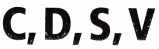# Texas Go Math Grade 4 Lesson 13.5 Answer Key Line Symmetry

Refer to our Texas Go Math Grade 4 Answer Key Pdf to score good marks in the exams. Test yourself by practicing the problems from Texas Go Math Grade 4 Lesson 13.5 Answer Key Line Symmetry.

## Texas Go Math Grade 4 Lesson 13.5 Answer Key Line Symmetry

Essential Question

How can you check if a figure has line symmetry?

Unlock the ProblemOne type of symmetry found in geometric figures is line symmetry. This sign is in the hills above Hollywood, California. D0 the letters in the Hollywood sign show line symmetry?

A figure has line symmetry fit can he folded about a line so that its two parts match exactly.
A fold line, or a line of symmetry, divides a figure into two parts that are the same size and shape.

Activity Explore line symmetry.

Materials

• pattern blocks
• scissors
• tracing paper

Math Idea(A) Does the letter W have line symmetry?

STEP 1 Use pattern blocks to make the letter W.STEP 2 Trace the letterSTEP 3 Cut out the tracing.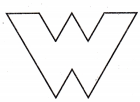STEP 4 Fold the tracing over a vertical line.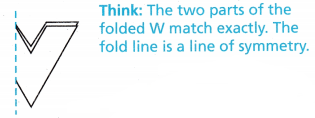So, the letter W ____________ line symmetry.
one line of symmetry

Explanation:
As we fold the paper the two parts of the folded W match exactly. Hence fold line is a line of symmetry. Therefore W has one line of symmetry.

Math Talk

Mathematical Processes
Why is it important to use a fold line to check if a figure has line symmetry?
A line that cuts a shape exactly in half. That means if you were to fold the shape along the line, both the halves would match exactly. Then we can tell that the fold line is a line of symmetry.

(B) Does the letter L have line symmetry?

STEP 1
Use pattern blocks or grid paper to make the letter L.STEP 2
Trace the letter.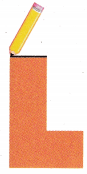STEP 3
Cut out the tracing.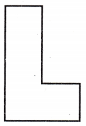STEP 4
Fold the tracing over a vertical line.STEP 5
Then open it and fold it horizontally.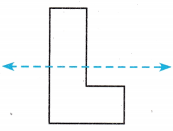STEP 6
Then open it and fold it diagonally.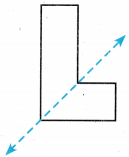So, the letter L _____________ line symmetry.
The letter L has no line of symmetry.

Explanation:
The given letter L has no line of symmetry though it was folded horizontally or vertically.

Math Talk

Mathematical Processes
Explain how you can use paper folding to check if a figure has line symmetry.
To check the line of symmetry, we have to fold the paper through the line. If the shapes on both sides of the line get matched, then the line through which the paper is folded can be considered as a line of symmetry.

Share and Show

Tell whether the parts on each side of the line match.
Is the line a line of symmetry? Write yes or no.

Question 1.Yes

Explanation:
We can imagine the above shape given as a paper. If we fold the paper through the dotted line, transparently the shapes on both sides of the line get matched. Hence the dotted line is a line of symmetry.

Question 2.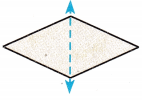Yes

Explanation:
We can imagine the above shape given as a paper. If we fold the paper through the dotted line, transparently the shapes on both sides of the line get matched. So, the dotted line is a line of symmetry.

Question 3.Yes

Explanation:
The given figure has a line of symmetry.

Question 4.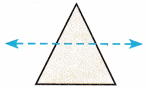No

Explanation:
We can imagine the above shape given as a paper. If we fold the paper through the dotted line, transparently the shapes on both sides of the line do not get matched. So, the given figure has no line of symmetry.

Problem Solving:

Complete the design by reflecting over the line of symmetry.

Question 5.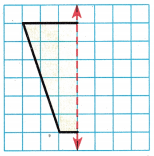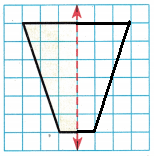Question 6.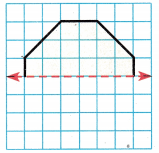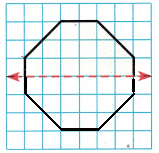Question 7.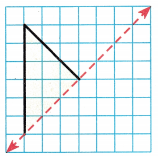Question 8.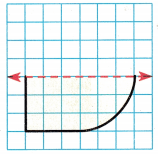Unlock the Problem

Question 9.H.O.T. Multi-Step Which figure has a correctly drawn line of symmetry?
(A)(B)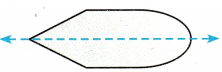(C)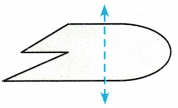(D)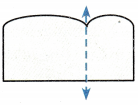a. What do you need to find?
To check whether the figure has a correctly drawn line of symmetry

b. How can you tell if the line of symmetry is correct?
If the shapes on both sides of the line get matched, then the line through which the figure has folded can be considered as a line of symmetry.

c. Tell how you solved the problem.

d. Fill in the bubble for the correct answer choice above.
Option B

Question 10.
Use Diagrams In which figure does the blue line appear not to be a line of symmetry?
(A)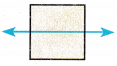(B)(C)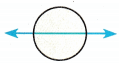(D)Option B

Explanation:

Lesson 13.5 Numbers with Lines of Symmetry Answer Key Question 11.
Casey is using foam stickers in different shapes to make a design. In which figure does the blue line appear to be a line of symmetry?
(A)(B)(C)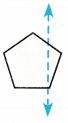(D)Option B

Explanation:
The figure given in option B has one line of symmetry.

Question 12.
Riley is making a pattern using trapezoids. In which trapezoid does the blue line appear to be a line of symmetry?
(A)(B)(C)(D)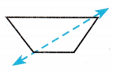Option B

TEXAS Test Prep

Question 13.
Which best describes the line of symmetry in the letter M?(A) horizontal
(B) diagonal
(C) vertical
(D) rotational
Vertical

### Texas Go Math Grade 4 Lesson 13.5 Homework and Practice Answer Key

Tell whether the parts on each side of the line match.
Is the line a line of symmetry? Write yes or no.

Question 1.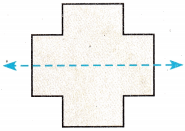Yes

Question 2.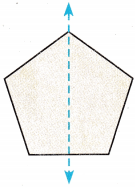Yes

Question 3.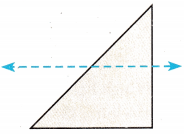No

Question 4.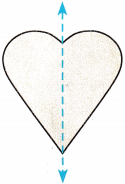Yes

Problem Solving

Complete the design by reflecting over the line of symmetry.

Question 5.Question 6.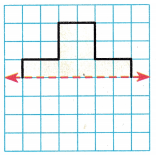Lesson Check

Question 7.
In which figure does the blue line appear to be a line of symmetry?
(A)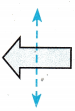(B)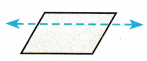(C)(D)Option C

Explanation:
The figure from option C has a line of symmetry.

Practice and Homework Lesson 13.5 Answer Key Question 8.
Which of the letters has line symmetry?
(A)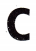(B)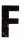(C)(D)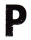Option A

Explanation:
The letter C from Option A has line symmetry.

Question 9.
Nora is making a pattern using a star shape. In which star does the blue line appear to be a line of symmetry?
(A)(B)(C)(D)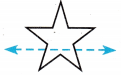Option B

Explanation:
The star does the blue line appear to be a line of symmetry is Option B.

Question 10.
Multi-Step Which best describes the symmetry in the letter I?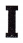(A) vertical only
(B) horizontal only
(C) vertical and horizontal
(D) diagonal and vertical
Horizontal only.

Explanation:
The letter I have horizontal symmetry only.

Question 11.
Multi-Step Which group of letters has no line of symmetry?
(A)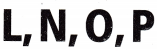(B)(C)(D)